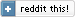(October 2007)

## Computing permutations

What is a permutation? Reading from my local copy of Wikipedia:
Permutation is the rearrangement of objects or symbols into distinguishable sequences. Each unique ordering is called a permutation. For example, with the numerals one to six, each possible ordering consists of a complete list of the numerals, without repetitions. There are 720 total permutations of these numerals, one of which is: "4, 5, 6, 1, 2, 3".

How do you go about calculating all possible permutations of a set? Well, if you know a priori the size of the input set, you can just write as many loops as is the set's cardinality. If, for example, we only have to deal with sets of 4 elements, ...

```for a in (1,2,3,4):
for b in (1,2,3,4):
if b == a: continue  # No repetitions allowed
for c in (1,2,3,4):
if c in [a,b]: continue  # No repetitions allowed
for d in (1,2,3,4):
if d in [a,b,c]: continue  # No repetitions allowed
print values[a], values[b], values[c], values[d]
```

That's all fine and simple, but what if you don't know in advance how large the set is?
You have to perform the same kind of loops, only you don't know how deep you'll have to go.

"Deep"?

Whenever "deep" comes up, recursion is knocking on the door...

```def permute(inputData, outputSoFar=[]):
outputSizeToReach = len(inputData)
for elem in inputData:
if elem not in outputSoFar:
outputSoFar.append(elem)
if len(outputSoFar) == outputSizeToReach:
print outputSoFar
else:
permute(inputData, outputSoFar)  # --- Recursion
outputSoFar.pop()

permute([1, 2, 3])
```
This prints:
```\$ python simple.py
[1, 2, 3]
[1, 3, 2]
[2, 1, 3]
[2, 3, 1]
[3, 1, 2]
[3, 2, 1]```
If you look at the code of permute, you'll again see the core loop iterating over all our input data: for elem in inputData. Since we don't know how deep we'll have to go - that is, how many "slots" exist that we will have to run this loop for - we will use the same loop for all data "slots", through recursion. In each step of the recursion, we first verify that the data hasn't been used yet (remember, permutations don't have repetitions, and we are assuming here that all our inputData are unique), then put the element inside outputSoFar, and recurse to proceed with the next available slot. If we run out of available slots, we've just created a complete permutation, so we print outputSoFar.

Clean, simple code. But... It just prints the results. What if we want to take some action on each permutation?

We could of course pass a lambda or a function (for execution on each permutation) as an extra argument to permute... But there is a better, a much better way:

```def permute(inputData, outputSoFar=[]):
outputSizeToReach = len(inputData)
for elem in inputData:
if elem not in outputSoFar:
outputSoFar.append(elem)
if len(outputSoFar) == outputSizeToReach:
yield outputSoFar
else:
for v in permute(inputData, outputSoFar):
yield v
outputSoFar.pop()
```
And now, we just do...
```for i in permute([1,2,3]):
print i  # or whatever else you want to do with it
```
Isn't this the best possible interface for permutation enumeration? This time we yield the results, instead of printing them! Using only this change, we create a much clearer and elegant interface, that users of our function will surely appreciate.

In case you haven't met yield before: this requires no extra runtime memory! The iteration process uses the magic behind yield, which "freezes" the state (call stack, "instruction pointer", etc) when it returns the result, and continues from the exact place it last was, when the iterator protocol asks for the next value.

Note that the code above doesn't use any kind of library... It simply uses a language feature to implement what we need. Now try implementing the same permutation interface in plain C++, without using any library (STL permutations or otherwise) ... See why I love yield? :‑)

P.S. Notice that where the original permute function called itself (the recursive call) a slight modification had to be done: by invoking yield, the permute function is now a generator, so we can't just simply call it again - if we did, we would lose the returned sequence, and thus, the returned permutations! We must instead loop over the returned results with a simple for v in permute(...): yield v. This way, the results are properly propagated to the "parent" call.

Update: For people developing in C/C++, here is an excellent article by Simon Tatham on how he implemented coroutines (i.e. yield-like behaviour) in C.

Update, some years later: It's also important to know that there's a difference between how Python implements yield and how other languages do.

 Index  CV Updated: Sat Oct 8 12:33:59 2022

The comments on this website require the use of JavaScript. Perhaps your browser isn't JavaScript capable or the script is not being run for another reason. If you're interested in reading the comments or leaving a comment behind please try again with a different browser or from a different connection.